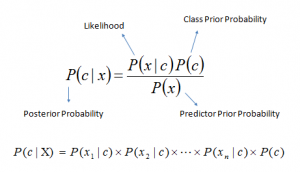#.## Introduction

Here’s a situation you’ve got into:

You are working on a classification problem and you have generated your set of hypothesis, created features and discussed the importance of variables. Within an hour, stakeholders want to see the first cut of the model.

What will you do? You have hunderds of thousands of data points and quite a few variables in your training data set. In such situation, if I were at your place, I would have used ‘Naive Bayes‘, which can be extremely fast relative to other classification algorithms. It works on Bayes theorem of probability to predict the class of unknown data set.

In this article, I’ll explain the basics of this algorithm, so that next time when you come across large data sets, you can bring this algorithm to action. In addition, if you are a newbie in Python, you should be overwhelmed by the presence of available codes in this article.

##1. What is Naive Bayes algorithm?
2. How Naive Bayes Algorithms works?
3. What are the Pros and Cons of using Naive Bayes?
4. 4 Applications of Naive Bayes Algorithm
5. Steps to build a basic Naive Bayes Model in Python
6. Tips to improve the power of Naive Bayes Model